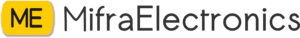# Interface Ultrasonic sensor with Arduino Uno

Ultrasonic sensor is one of the main part of numerous projects. Here we are going to interface a Ultrasonic sensor HC-SR 05 with Arduino Uno.

In this project we discuss,

• Connect a Ultrasonic Sensor HC-SR 05 to Arduino.
• Read the sensor and convert it to length.
• Print the length to the Serial Monitor.

### HC – SR 05

Before starting we need to know about the working of Ultrasonic Sensor HC-SR 05. Actually it consist of an ultrasonic transmitter and an ultrasonic reciver. First ultrasonic transmitter send an ultrasonic wave. This signal will collide with the object and reflect the signal. The receiver will receive the reflected signal. The distance calculated by the time taken to receive the reflected. and the speed of sound in air. The speed of sound in air at room temperature is 340 Meter/Second or 0.034 centimeter/microsecond. The equation for calculating time is,

Time=Distance/speed of sound

If the object is 10 CM away from the sensor, You will get the time as per the equation is,

10/0.034 = 294.11 Microseconds

But you will get the value from the Echo pin is 588.22. This is because of the sound wave needs to travel forward and bounce backward. So we need to devide that value by 2 for get the actual value(time). Here we want to calculate the distance from the time. So re-arrange the equation we will get,

Distance=Time x speed of sound

The time to start.

Buy electronic components with free shipping on mifraelectronics.com

Step – 1

Open the arduino software at mifratech youtube channel (refer my previous article for introduction to Arduino IDE here) and we need define two pins, echoPin on digital pin 2 and trigPin on digital pin 3. by using the keyword “define’. Next declare two variables, one is “duration”. This is for store the duration of sound wave traveled. Other is “distance” for store the distance calculated.

``#define echoPin 2#define trigPin 3long duration;int distance;``

definition part is completed. Next setup part.

Step – 2

In the void setup() function we need to begin the serial communication with baurd rate as 9600. It is done by the keyword “Serial.begin(9600)”. Then set the trigPin as “OUTPUT”, by the keyword “pinMode(trigPin, OUTPUT)”. Because the trigPin is the input pin of transmitter of sensor module. Now we need to set the echoPin as “INPUT”. By the keyword “pinMode(echoPin, INPUT)”.

``void setup(){  Serial.begin(9600);  pinMode(trigPin,OUTPUT);  pinMode(echoPin,INPUT); }``

setup part is completed.

Now we need to code the loop part.

Step – 3

Now the trigPin state is in a float condition. We need to set it as “LOW”. for this purpose we use the keyword “digitalWrite(trigPin, LOW)”. Then hold this state for 2 microseconds by the keyword “delayMicroseconds(2)”

``digitalWrite(trigPin,LOW);delayMicroseconds(2);``

Now we need to set the trigPin “HIGH” for 10 seconds, with the same keyword mentioned above. Only change the parameter.

``digitalWrite(trigpin,HIGH);delayMicroseconds(10);``

Then set the trigPin as “LOW” state.

``digitalWrite(trigpin,LOW);``

Now read the echoPin and put it to the function “pulseIn(echoPin, HIGH)”. This returns the total travel time. So we need to store this return value to the variable “duration”.

``duration=pulseIn(echoPin,HIGH);``

The total travel time is now stored in the variable “duration”

Now we can calculate the distance from this duration by using the equation. And store calculated value(distance) to the variable “distance”. The eqution is explained above

``distance=(duration*0.034/2);``

The distance from the sensor to the object is now stored in the variable “distance”.

Then we need to display it to screen. For this purpose, here we using the serial communication. Ypu can also use LCD, Sven Segment Display, OLED Disply, etc…(The will change). First print a headingor a message. Here I am going to print “Distance”. by using “Serial.print(“Distance : ” )”. After that print the distance to the serial monitor, we use the keyword “Serial.println(distance)”. Then print the unit by “Serial.println(” cm “)”. Here I used a “ln” with “Serial.print()”. It’s for start a new line. The code is like,

``Serial.print("Distance : " );Serial.print(distance)";Serial.println(" cm ")";``

The code is completed. Upload the code to Arduino Uno. The complete code is given in the code part.

Step – 4

Next add a 1 second delay

``delay(1000);``

Step – 5

Then connect the Ultrasonic sensor HC -SR05 to Arduino Uno with female to male jumber wire.

HC -SR05 Arduino Uno

Vcc 5V

echo D2

trig D3

GND GND

Step – 5

Now we need to open Serial monitor from top right corner of Arduino IDE. Please ensure the selected COM port(in Windows OS) is right and braud rate is 960